## Fibonacci Day

Now on Display in the Math Library: A Celebration of Fibonacci Day!

11/23 is Fibonacci Day!
The first four numbers of the Fibonacci sequence are 1 1 2 3 (therefore we celebrate on 11/23).

What is the Fibonacci Sequence?

The Fibonacci sequence is a sequence of numbers.  Add the last two numbers in the sequence to get the next number.  The first six numbers of the sequence are 1 1 2 3 5 8, so:
1
1+0=1
1+1=2
1+2=3
2+3=5
3+5=8

And this can go on forever!

Draw boxes using the Fibonacci sequence…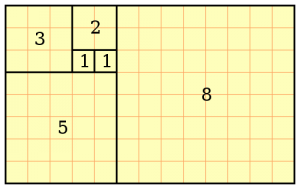and draw a curve through the diagonals of each box…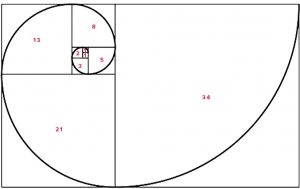VOILA! A Fibonacci Spiral!

All sorts of natural phenomena follow the Fibonacci spiral.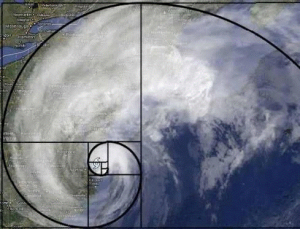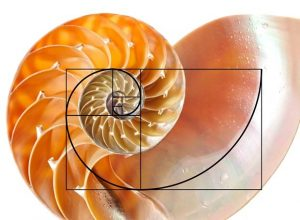Even our pets can get in on the fun!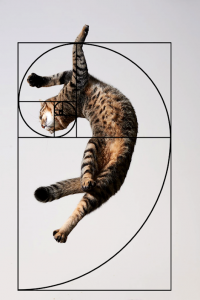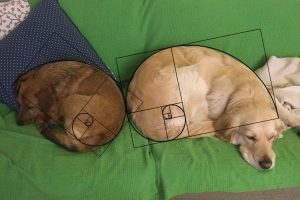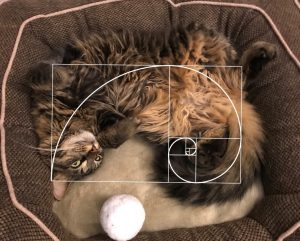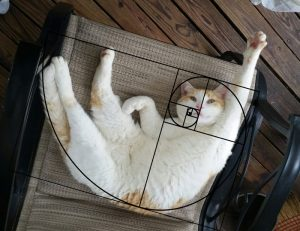Books on Display:

Nature’s numbers : the unreal reality of mathematical imagination / Ian Stewart. (Math 510 ST492N)

The Golden ratio and Fibonacci numbers / by Richard A. Dunlap. (Math 512.72 D921g1997)

Mathematics in nature : modeling patterns in the natural world / John A. Adam. (Math 511.8 Ad13m)

Mathematics in nature : modeling patterns in the natural world / John A. Adam. (E-Book)

Fabulous Fibonacci numbers / Alfred S. Posamentier, Ingmar Lehmann ; afterword by Herbert A. Hauptman. (Math 512.72 P84f)

Finding Fibonacci : the quest to rediscover the forgotten mathematical genius who changed the world / Keith Devlin. (Math 510.92 F44d)

New visual perspectives on Fibonacci numbers / K.T. Atanassov [and others]. (Math 512.72 N42)

Wild Fibonacci : nature’s secret code revealed /by Joy N. Hulme ; illustrations by Carol Schwartz. (SSHEL S.512.72 H878w)

A special thanks to Kitty Garner, Grainger Engineering Library Graduate Assistant, for creating this Mathematics Library exhibit.

Updated on